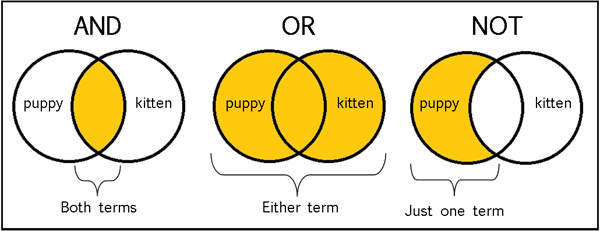Related Tags

pascal
boolean operators

# What are boolean operators in Pascal?

In this shot, let’s talk about Boolean operators in Pascal. We often use these boolean operators in condition variables.

 Operator How does it work Condition Statement and This refers to the AND operator often used in boolean calculations. It gives output True if both inputs A and B are True. Output = True IF (A = True and B = True) and then This is similar to the AND operator often used in boolean calculations. It is a conditional AND, where the second input B is only checked if first input A is True. It gives output True if both inputs A and B are True. This condition is used to clarify the order of execution of condition statements, left to right. Output = True IF (A = True and then B = True) or This refers to the OR operator often used in boolean calculations. It gives output True if any of the inputs A or B are True. Output = True IF (A = True or B = True)Output = True IF (A = True or B = False)Output = True IF (A = False or B = True) or else This is similar to the OR operator often used in boolean calculations. It is a conditional OR, where the second input B is only checked if first input A is False. It gives output True if both any of inputs A or B are True. This condition is used to clarify the order of execution of condition statements, left to right. Output = True IF (A = False or else then B = True) not This refers to the OR operator often used in boolean calculations. It is used to reverse the initial value of any input. output = False if not Aoutput = False if not Boutput = true if (not A or B)## Code

program beLogical;
var a, b : boolean;
// initialise the boolean values a and b
begin
// declare both of them as True initially
a := true;
b := true;

// If both are True, AND will result in True.

// This means that condition will be true overall.

if (a and b) then
writeln('Condition And is True');

// If both are True, OR will result in True.

// This means that condition will be true overall.

if  (a or b) then
writeln('or condition is True');

end.


Output:

Condition And is True
or condition is True


RELATED TAGS

pascal
boolean operators

CONTRIBUTOR